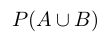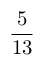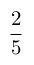Select Page

# Solutions for MCQ CBSE 12 Science Maths Vector Algebra in English

Solutions for MCQ CBSE 12 Science Maths Vector Algebra in English to enable students to get Solutions in a narrative video format for the specific question.

Expert Teacher provides Solutions for MCQ CBSE 12 Science Maths Vector Algebra through Video Solutions in English language. This video solution will be useful for students to understand how to write an answer in exam in order to score more marks. This teacher uses a narrative style for a question from Vector Algebra not only to explain the proper method of answering question, but deriving right answer too.

Please find the question below and view the Solution in a narrative video format.

Question:

Solution Video in English:

You can select video Solutions from other languages also. Please check Solutions in ( Hindi )

## Similar Questions from CBSE, 12th Science, Maths, Vector Algebra

Question 1 :  Find the direction cosines of the vector joining the points A(1, 2, -3) and B(-1, -2, 1) directed from B to A. (View Answer Video)

Question 2 : Find the unit vector in the direction of vector PQ, where P and Q are the points (1, 2, 3) and (4, 5, 6) respectively. (View Answer Video)

Question 3 : Find the area of the triangle with vertices A(1, 1, 2), B(2, 3, 5) and C(1, 5, 5). (View Answer Video)

Question 4 : L and M are two points with position vectorsandrespectively. Write the position vectors of a point N which divides the line segment LM in the ratio 2:1 externally.   (View Answer Video)

Question 5 : Find the position vector of a point which divides the join of points with position vectorsandexternally in the ration 2:1.  (View Answer Video)

### Probability

Question 1 : An insurance company insured 2,000 cyclists, 4,000 scooter drivers and 6,000 motorbike drivers. The probability of an accident involving a cyclist, scooter driver and a motorbike driver are 0.01, 0.03, and 0.15 respectively. One of the insured persons meets with an accident. What is the probability that he is a scooter driver?     (View Answer Video)

Question 2 : A speaks truth in 75% of the cases, while B in 90% of the cases. In what percent of cases are they likely to contradict each other in stating the same fact? Do you think that statement of B is always true?     (View Answer Video)

Question 3 : Evaluate, if 2P(A)=P(B)=and=. (View Answer Video)

Question 4 : A speaks truth in 60% of the cases, while B in 90% of cases. In what percent of cases are they likely to contradict each other in stating the same fact? In the cases of contradiction do you think, the statement B will carry more weight as he speaks truth in more number of cases than A?    (View Answer Video)

Question 5 : A class has 15 students whose ages are 14, 17, 15, 14, 21, 17, 19, 20, 16, 18, 20, 17, 16, 19 and 20 years. One student is selected in such a manner that each has the same chance of being chosen and the age X of the selected student is recorded. What is the probability of the random variable X? Find the mean.   (View Answer Video)

### Matrices

Question 1 : If, then find "a". (View Answer Video)

Question 2 : Matrices A and B will be inverse of each other only if : (View Answer Video)

Question 3 : Find the transpose of the matrix:. (View Answer Video)

Question 4 : Find the value of y, from the equation:(View Answer Video)

Question 5 : Given,, find the value of z. (View Answer Video)

### Three Dimensional Geometry

Question 1 : A line passes through the point with position vectorand makes anglesandwith x, y and z-axis respectively. Find the equation of the line in the Cartesian form. (View Answer Video)

Question 2 : Find the co-ordinates of the point where the line through the points (3, -4, -5) and (2, -3, 1) crosses the plane
Question 4 : Find the distance of the point (-1, -5, -10) from the point of intersection of the lineand plane(View Answer Video)